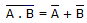# Electronics - Logic Circuit Simplification

### Exercise :: Logic Circuit Simplification - True or False

1.

Subtraction is commutative.

 A. True B. False

Explanation:

No answer description available for this question. Let us discuss.

2.

The expressions,, are equivalent.

 A. True B. False

Explanation:

No answer description available for this question. Let us discuss.

3.

The Boolean expression for a three-input AND gate is Y = A • B + C.

 A. True B. False

Explanation:

Y = A • B • C

4.

According to the commutative law, in ORing and ANDing of two variables, the order in which the variables are ORed or ANDed makes no difference.

 A. True B. False

Explanation:

No answer description available for this question. Let us discuss.

5.

Boolean multiplication is symbolized by A + B.

 A. True B. False